# Basis of a Vector Space Example

A set of vectors in vector space V is called a basis for V if the vectors in the set are linearly independent and every vector is a linear combination of vectors in this set. The former one equals the vector space being spanned by the set. The dimension of vector space V is the number of vectors in its basis. A vector space is called nite dimensional if it has a nite basis.

Example 1. Let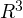be the real vector space in three dimensions, and consider the set of vectors S=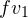;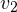;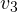g where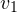= (1; 2; 0),= (0; 1; 0) and= (1; 0; 1). Let us see whether S is a basis foror not.

S is linearly independent since the solution for the equation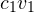+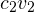+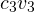= 0 is unique, and it is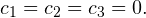On the other hand, we need to investigate whether the vectors span the whole vector space. Any vector inis of the form (a; b; c). We need to end coefficients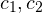and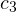in terms a, b and c.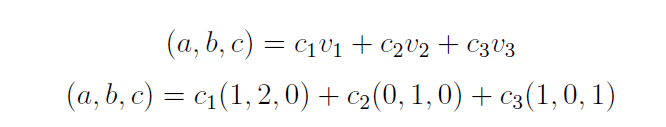Once we apply Gauss-Jordan reduction to this equation we get the following solution: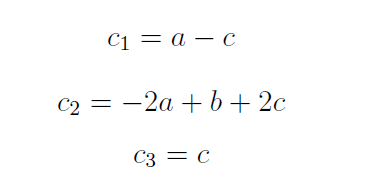The standard basis foris f(1; 0; 0); (0; 1; 0); (0; 0; 1)g. The dimension ofis three, since there are three vectors in its bases.

Example 2. Now, consider the set of vectors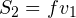;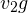where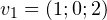and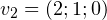and consider another set of vectors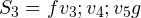where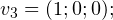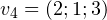and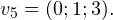The first set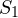is not a basis forsince it has two vectors, and a set of two vectors cannot span a vector space with dimension three. The second set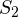is not a basis forbecause it is not linearly independent, since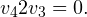This sample will help you deal with the basis of a vector sector. You will spend much less time on your assignment with the help of the sample. Of course, this basis of a vector sector example was created by an expert who is knowledgeable in the topic. Therefore, you can be sure that it is absolutely correct.